# Rational (Fractional) Exponents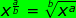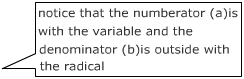Now let's practice with a few numerical expressions.

•••A little more challenging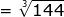write as a radical and simplify

=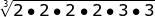expand using prime factorization

=group triples because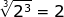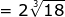simplify

Now let's practice with a few variable expressions.

•This is a little more challenging because the numerator is larger than the denominator.

•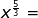•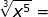•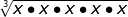=
expand

•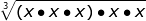=

group triples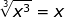and you still have x2

•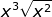An easy way to remember the rule is to choose an example that you know for sure. For example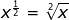. This way you can remember that the denominator is the number that goes with the radical and the numerator goes with the number/variable under the radical.

Reminder: the exponent rules do not change when you are multiplying, dividing or raising a power to a power just because the exponent is a fraction.

••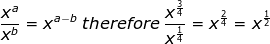•Related Links: Math algebra Exponents Exponents and Powers The Laws of Exponents Integers as Exponents Evaluate Exponents Evaluate integers with exponents Positive and Negative Integer Exponents Rational (Fractional) Exponents Zero Exponents Dependent and Independent Variables

To link to this Rational (Fractional) Exponents page, copy the following code to your site: